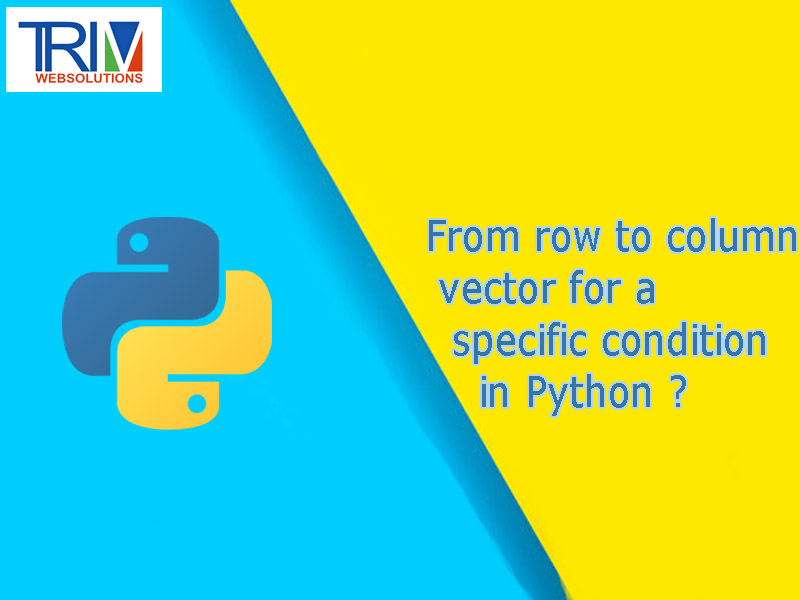Quick Inquiry

# From row to column vector for a specific condition in python ?You can use this:

` `values = [[x] for x in values] ``

and make it a numpy array like this:

``` ```values = Flux[Flux >= 0]
values = np.array([[x] for x in values])
print("positive values =",[values])``````